The Hurst ExponentBy Varun Divakar and Ashish Garg

In this blog, we will be discussing an important concept in time series analysis: The Hurst exponent. We will learn how to calculate it with the help of an example. First, let us understand what Hurst exponent is.

Hurst Exponent Definition

The Hurst exponent is used as a measure of long-term memory of time series. It relates to the autocorrelations of the time series and the rate at which these decrease as the lag between pairs of values increases.

Hurst Value

Hurst Value is more than 0.5

If the Hurst value is more than 0.5 then it would indicate a persistent time series (roughly translates to a trending market).

Hurst Value is less than 0.5

If the Hurst Value is less than 0.5 then it can be considered as an anti-persistent time series (roughly translates to sideways market).

Hurst Value is 0.5

If the Hurst value is 0.5 then it would indicate a random walk or a market where prediction of future based on past data is not possible.

How To Calculate The Hurst Exponent

To calculate the Exponent, we need to divide the data into different chunks. For example, if you have the return data of BTC/USD for the past 8 days’ data, then you divide it into halves as follows: Following the example of 8 observations for illustrative purposes only1: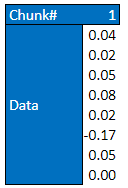1Length of the subseries in practical applications is usually much longer and affects the mean and standard deviation of the R/S statistic.

Then we divide the data into 3 different chinks as follows:

1. Division 1 - one chunk of 8 observations
2. Division 2 - two chunks of 4 observations each
3. Division 3 - four chunks of 2 observations each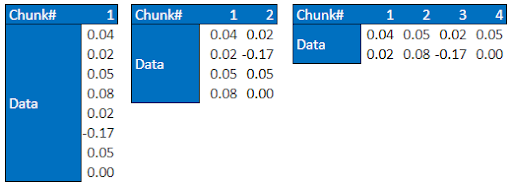After dividing the data into chunks, we perform the following calculations on each chunk:

1. First we calculate the mean of the chunk, with say n observations,

M = (1/n) [ h(1)+h(2)+...+h(n) ]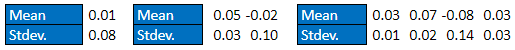1. Then we calculate the standard deviation (S) of the n observations
s(n) = STD( h(1)+h(2)+...+h(n))1. Then we create a mean centered series by subtracting the mean from the observations,
x(1) = h(1) - M

x(2) = h(2) - M

...

x(n) = h(n) - M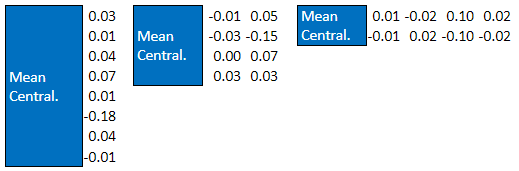Then we calculate the cumulative deviation by summing up the mean centred values,

Y(1) = x(1)

Y(2) = x(1) + x(2)

...

Y(n) = x(1) + x(2) + ...+ x(n)

5. Next, we calculate the Range (R), which is the difference between the maximum value of the cumulative deviation and the minimum value of the cumulative deviation,

R(n) = MAX[Y(1),Y(2)...Y(n)] - MIN[Y(1),Y(2)...Y(n)]
1. And finally, we compute the ratio of the range R to the standard deviation S. This also known as the rescaled range.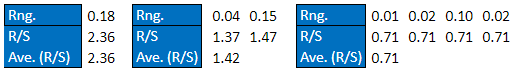Once we have the rescaled range for all the chunks, we compute the mean of each Division and note it along with the number of samples in each chunk of that Division as shown.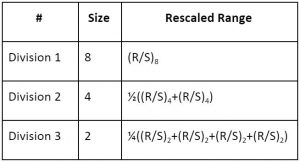Next, we calculate the logarithmic values for the size of each region and for each region’s rescaled range.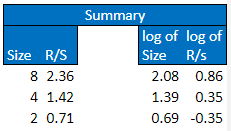The Hurst exponent ‘H’ is nothing but the slope of the plot of each range’s log(R/S) versus each range’s log(size). Here log(R/S) is the dependent or the y variable and log(size) is the independent or the x variable: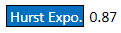This hurst exponent value is indicating that our data is a persistent one, but we have to keep in mind that our data set is too small to draw such a conclusion. For example, if you want to calculate hurst exponent in python using the ‘hurst’ library, it requires you to give at least 100 data points.

We hope you have learnt how to calculate the Hurst exponent from this blog. In our advanced course on cryptocurrencies, we have demonstrated how hurst exponent along with another technical indicator can yield optimized trading signals.

Disclaimer: All investments and trading in the stock market involve risk. Any decisions to place trades in the financial markets, including trading in stock or options or other financial instruments is a personal decision that should only be made after thorough research, including a personal risk and financial assessment and the engagement of professional assistance to the extent you believe necessary. The trading strategies or related information mentioned in this article is for informational purposes only.